# KSEEB Solutions for Class 9 Maths Chapter 11 Areas of Parallelograms and Triangles Ex 11.2

In this chapter, we provide KSEEB SSLC Class 9 Maths Chapter 11 Areas of Parallelograms and Triangles Ex 11.2 for English medium students, Which will very helpful for every student in their exams. Students can download the latest KSEEB SSLC Class 9 Maths Chapter 11 Areas of Parallelograms and Triangles Ex 11.2 pdf, free KSEEB SSLC Class 9 Maths Chapter 11 Areas of Parallelograms and Triangles Ex 11.2 pdf download. Now you will get step by step solution to each question.

## Karnataka Board Class 9 Maths Chapter 11 Areas of Parallelograms and Triangles Ex 11.2

Question 1.
In Fig., ABCD is a parallelogram, AE ⊥ DC and CF ⊥ AD. AB = 16 cm., AE = 8 cm, CF = 10cm, find AD.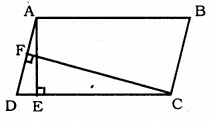Solution:
ABCD is a parallelogram.
AE ⊥ DC and CF ⊥ AD.
AB = 16 Cm, AE = 8 cm, CF = 10 cm, then AD = ?
(i) Area of ABCD = base × altitude
= DC × AE ( ∵ AB=DC)
= 16 × 8
= 128 sq. cm.
∴ Area of ADCB = 128 sq. cm.
Altitude, CF= 100 cm.
Base × Altitude = Area

Question 2.
If E, F, G and H are respectively the mid-points of the sides of a parallelogram ABCD, show that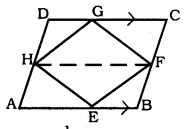ar(EFGH) = 12 ar(ABCD)
Solution:
Data: E, F, G and H are mid-points of the sides of a parallelogram ABCD,
To Prove: area (EFGH) = 12 area (ABCD)
Construction: HF is joined.
∴ 2AH = 2BF
∴ AH = BF and AH || BF
∴ AHFB is a parallelogram.
Similarly, HDCF is a parallelogram.
Now, DEHF and Quadrilateral AHFD are on base HF and in between HF || AB.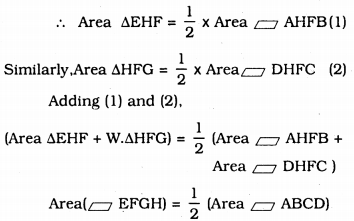Question 3.
P and Q are any two points lying on the sides DC and AD respectively of a parallelogram ABCD. Show that ar (PAB) = ar (BQC).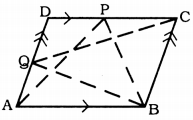Solution:
Data: P and Q are any two points lying on the sides DC and AD respectively of a parallelogram ABCD.
To Prove: ar(∆APB) = ar(∆BQC)
Proof: ABCD is a parallelogram.
∴ AB || DC AB = DC
Now ∆APB and ABCD are on same base AB and in between AB || DC
∴ Area(∆APB) = 12  Area ABCD) ……… (i)
Similarly, ∆BQC and BADC are on the same base BC and in between BC || AD.
∴ Area(∆BQC) = 12  Area(ABCD) ………. (ii)
From (i) and (ii),
∴ Area(∆APB) = Area (∆BQC).

Question 4.
In Fig., P is a point in the interior of a parallelogram ABCD, Show that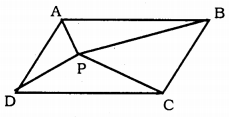(i) ar.(ABP)= ar(PCD) = 12 ar (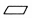ABCD)
(ii) ar(APD)+ ar(PBC) = ar(APB) + ar(PCD)
(Hint: Through P, draw a line parallel to AB).
Solution:
Data : P is any point in the interior of a parallelogram ABCD. PA, PB, PC and PD are joined.
To Prove: (i) ar(ABP= ar(PCD)= 12 ar (ABCD)
(ii) ar(APD) + ar(PBC)= ar(APB) + ar(PCD)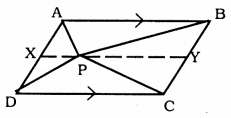Construction: AB || XY is drawn through P.
Proof: (i) XY || AB || DC
∴ ABYX and XDCY are parallelograms.
∆APB andABYX are on base AB and in between AB||XY.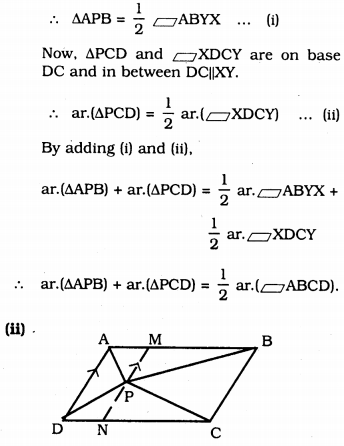Construction: AD || MN is drawn through P.
Proof: ∆ADP andADMN lie on the base AD and in between AD || MN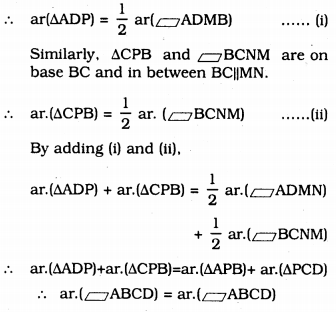Question 5.
In Fig., PQRS and ABRS are parallelograms and X is any point on side BR. Show that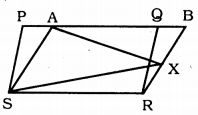(i) ar.(PQRS) = ar.(ABRS)
(ii) ar.(AXS) + = 12 ar. (PQRS).
Solution:
Data: PQRS and ABRS are parallelograms and X is any point on side BR.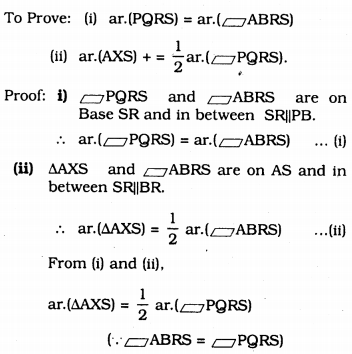Question 6.
A farmer was having a field in the form of a parallelogram PQRS. She took any point A on RS and joined it to points P and Q. In how many parts the fields is divided? What are the shapes of these parts? The farmer wants to sow wheat and pulses in equal portions of the field separately. How should she do it?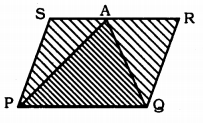Solution:
If AP and AQ are joinedPQRS is divided into three triangles, ∆SAP, ∆APQ and ∆ARQ.
∆APQ andPQRS are on base PQ and in between PQ || SR.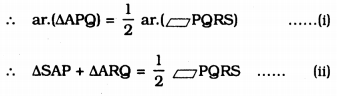From (i) and (ii).
∴ ar.(∆APQ) = ar.(∆SAP) + ar.(∆ARQ)
∴ Farmer can use the part of the field to sow wheat,
i.e. Ar. (∆SAP + ∆ARQ) and in the same area, he can use the Area of ∆APQ to sow pulses.

All Chapter KSEEB Solutions For Class 9 Maths

—————————————————————————–

All Subject KSEEB Solutions For Class 9

*************************************************

I think you got complete solutions for this chapter. If You have any queries regarding this chapter, please comment on the below section our subject teacher will answer you. We tried our best to give complete solutions so you got good marks in your exam.

If these solutions have helped you, you can also share kseebsolutionsfor.com to your friends.

Best of Luck!!